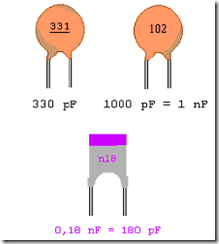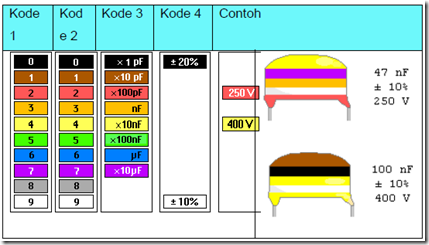" /> Encoding Capacitors | TN Elektro
Home > English > Encoding Capacitors

# Encoding Capacitors

Some capacitors issued by the factory has a way of encoding different. Broadly speaking encoding a dielectric capacitor can be sorted by type of material, the working voltage, capacitance value and its tolerance, whereas for the specification of the data that is more specific and detailed plant usually informed through the datasheet; such as the value of the series or parallel resistance, leakage current losses. To find out all the meaning that is written on the components of the capacitors, then it is very important to understand how the writing of the codes.

Encoding capacitance and tolerance, explaining the value of the capacitance of a capacitor with a given tolerance limits, usually writing code capacitor refers to the regulations IEC (International Elektrotechnik Commission), based on the results of testing at a temperature of 20ºC. For code writing tolerance is generally expressed in capital letters and written after the code capacitance value. There are two kinds of writing encoding capacitor, which is writing the alphabet and color code. One example, within the capacitor body writing the code as follows:

Encoding with numbers
Non polar capacitors are generally small capacitance value of the capacitors and the information encoded in the form of numbers for capacitance value and in the form of letters to tolerance, as an example image below there are several types of ceramic capacitors that provide information only capacitance aloneFigure 5.8 Example of coding on the capacitor

In the picture above ceramic capacitors are coded with three digits, which means Figures 1 = Score
Figures to 2 = Score and
Figures to 3 = Multiplication (number zero)
Sometimes there are only two numbers on the capacitor with a capacitance that is small, therefore these figures is the capacitance, the unit of the capacitor is expressed in units of pico Farat (pF)
Other examples of coding:
0.47 M 250:
0.47 The amount of capacitance of 0.47 μF
M The amount of tolerance of ± 20%
250 The amount of allowable working voltage of 250V

The working voltage, the limit of the maximum working voltage DC or AC voltage may be permitted at the maximum working temperature of 40ºC. For writing code working voltage is generally expressed with small letters or directly expressed in numbers and written after the code of tolerance.

Table 2.2 Example of coding element capacitor.Figure 5.9 Physical form of capacitors with color codes